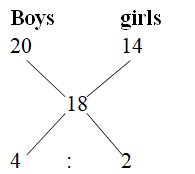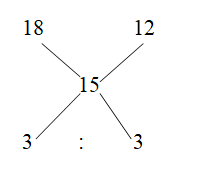# Quantitative Aptitude Questions (Inequality) for SBI Clerk / IDBI Executive 2018 Day- 77

Dear Readers, SBI is conducting Online preliminary Examination for the recruitment of Clerical Cadre. preliminary Examination of SBI Clerk was scheduled from June 2018. To enrich your preparation here we have providing new series of Inequality – Quantitative Aptitude Questions. Candidates those who are appearing in SBI Clerk Prelims Exam can practice these Quantitative Aptitude average questions daily and make your preparation effective.

[WpProQuiz 2113]

Click “Start Quiz” to attend these Questions and view Solutions

Directions (Q. 1-5) Each question below contains a statement followed by Quantity I and Quantity II. Find the relationship among them and mark your answer accordingly.

1. Quantity I: Marked price of an article, which is purchased for Rs. 650 and the shopkeeper allowed a discount of 20% on the article while the profit earned on the article is Rs. 62?

Quantity II: Selling price of an article, which is marked at Rs. 1250 in which shopkeeper allows two successive discounts of 10% and 15%?

a) Quantity I > Quantity II
b) Quantity I ≥ Quantity II
c) Quantity II > Quantity I
d) Quantity II ≥ Quantity I
e) Quantity I = Quantity II or Relation cannot be established

1. There are 240 students in a class whose average age is 18 years. The average age of the boys in the class is 20 years while the average age of the girls is 14 years.

Quantity I: No of boys in the class is?

Quantity II: No of girls in the class is?

a) Quantity I > Quantity II
b) Quantity I ≥ Quantity II
c) Quantity II > Quantity I
d) Quantity II ≥ Quantity I
e) Quantity I = Quantity II or Relation cannot be established

1. A person invested a sum of Rs. 35600 in two schemes A and B which offers interest at the rate of 18% and 12 % respectively. If the total interest received from these schemes after 4 years is Rs. 5340 then,

Quantity I: Sum of money invested in scheme A

Quantity II: Sum of money invested in scheme B

a) Quantity I > Quantity II
b) Quantity I ≥ Quantity II
c) Quantity II > Quantity I
d) Quantity II ≥ Quantity I
e) Quantity I = Quantity II or Relation cannot be established

1. A sum of Rs. 2385 was divided among A, B and C in such a way that the amount received by A is 20% more that of C and the amount received by C is 25% less than the amount received by B.

Quantity I: Amount received by A.

Quantity II: Amount received by B.

a) Quantity I > Quantity II
b) Quantity I ≥ Quantity II
c) Quantity II > Quantity I
d) Quantity II ≥ Quantity I
e) Quantity I = Quantity II or Relation cannot be established

1. 12 boys and 16 girls can do a piece of work in 34 days, while 10 boys and 14 girls can do the same piece of work in 40 days.

Quantity I: In how many days 20 boys and 14 girls will complete the work?

Quantity II: In how many10 boys and 25 girls will complete the work?

a) Quantity I > Quantity II
b) Quantity I ≥ Quantity II
c) Quantity II > Quantity I
d) Quantity II ≥ Quantity I
e) Quantity I = Quantity II or Relation cannot be established

1. Quantity I: The profit earned by selling an article for Rs. 210 is 20% while the discount percentage allowed on the article is at the rate of 30% then find the percentage by which markup price of the article is raised above cost price?

Quantity II: What is the profit percentage earned by selling an article which costs Rs. 180 and the shopkeeper sold the article for Rs.320?

a) Quantity I > Quantity II
b) Quantity I ≥ Quantity II
c) Quantity II > Quantity I
d) Quantity II ≥ Quantity I
e) Quantity I = Quantity II or Relation cannot be established

1. Quantity I: The average age of five members in a family six years ago was 35 years while the present average age of 6 member is 39 years, daughter-in- law being the new member, then find the age of the daughter-in –law?

Quantity II: The ratio of ages of A and B at present is 2:3. Five years hence, their ages will be in the ratio 5 : 7 then find the present age of A?

a) Quantity I > Quantity II
b) Quantity I ≥ Quantity II
c) Quantity II > Quantity I
d) Quantity II ≥ Quantity I
e) Quantity I = Quantity II or Relation cannot be established

1. Quantity I: Simple Interest earned on a certain sum of money after 4 years is Rs. 2240 at the rate of 5% then find the principal?

Quantity II: Money invested by Rohan is Rs. x while the money invested by Sohan is (x – 4000) while the total the total interest received by Rohan and Mohan is Rs. 4800 at a simple interest rate of 4% after 6 years then find the value of x?

a) Quantity I > Quantity II
b) Quantity I ≥ Quantity II
c) Quantity II > Quantity I
d) Quantity II ≥ Quantity I
e) Quantity I = Quantity II or Relation cannot be established

1. Quantity I: A, B and C can complete a work in 6 days while A and B can the same work working alone in 20 and 35 days then find in how many days C can complete the work by working alone with ¾ of his original efficiency?

Quantity II: 15 men can complete in 20 days or 18 women can complete the same work in 24 days then find in how many days 10 men and 14 women can complete the same work?

a) Quantity I > Quantity II
b) Quantity I ≥ Quantity II
c) Quantity II > Quantity I
d) Quantity II ≥ Quantity I
e) Quantity I = Quantity II or Relation cannot be established

1. Quantity I: 180 m long train crosses an electric pole in 12 seconds then find the time taken by the same train to crosses a platform of length 230m?

Quantity II: Another train of length 120m crosses a man walking at a speed of 4 km/hr in the opposite direction of the train in 10 seconds then find the time taken by the train to cross a bridge of length 140m?

a) Quantity I > Quantity II
b) Quantity I ≥ Quantity II
c) Quantity II > Quantity I
d) Quantity II ≥ Quantity I
e) Quantity I = Quantity II or Relation cannot be established

Directions (Q. 1-5)

Explanation:

Quantity I:

Selling price of the article= 650 + 62 = 712

Marked price of the article= 712*100/80= Rs. 890

Quantity II:

Two successive discount

= 10+15- 10*15/100

= 25 – 1.5

= 23.5

Selling price= 1250*(76.5/100) = Rs. 956.25

Quantity II > Quantity I

Explanation:

Let the number of boys and girls in the class be x and y respectively

So,

X + y= 240……….(i)

20x + 14y= 240*18

20x+ 14y= 4320

By solving we get,

x= 160, y= 80

(Or)= > 2 : 1

3’s = 240

1’s = 80

Quantity I: Boys= 160

Quantity II: Girls= 80

Quantity I > Quantity II

Explanation:

Percentage of interest received from these schemes

= 5340*100/35600

= 15%

By the rule of allegation,= > 1 : 1

2’s = 35600

1’s = 17800

Quantity I: Sum of money invested in scheme A= Rs. 17800

Quantity II: Sum of money invested in scheme B = Rs. 17800

Quantity I = Quantity II

Explanation:

Let the amount received by B be 100

C’s share= 75

A’s share= 90

Ratio between the share of A: B: C= 90:100:75= 18:20:15

Quantity I: Share of A= 18*2385/53= Rs. 810

Quantity II: Share of B= 20*2385/53= Rs. 900

Quantity II > Quantity I

Explanation:

By the rule of M.D.H,

(12B + 16G)*34= (10B+14G)*40

408B+ 544G = 400B+560G

8B= 16G

B/G= 16/8= 2/1

Total work= (12*2+ 16*1)*34= 40*34= 1360 units

Quantity I:

Time required by 20 boys and 10 girls

Units of work done by 20 boys and 14 girls in one day

= 20*2+ 14*1= 54 units

Time required= 1360/54= 25 (5/27) days

Quantity II:

Units of work done by 10 boys and 25 girls in one day= 10*2+ 25*1= 45 units

Time required= 1360/45= 272/9= 30 (2/9) days

Quantity II > Quantity I

Explanation:

Quantity I:

Cost price = 210*100/120= Rs. 175

Marked price= 210*100/70= Rs. 300

Markup percentage= (300-175/175)*100 = 71.42%

Quantity II:

Cost price = Rs. 180

Selling price = Rs. 320

Profit percentage= (320-180/180)*100= 77.77%

Quantity II > Quantity I

Explanation:

Quantity I:

Total age of the family six years ago= 35*5= 175 years

Total ages of the family at present without daughter- in – law

= 175+ 5*6= 205 years

Total ages of the family now including daughter in law

= 39*6= 234 years

Age of the daughter in law= 234-205= 29 years

Quantity II:

Let the ratio be 2x and 3x

So,

2x+5/3x+5= 5/7

14x+35= 15x + 25

x= 10

Present age of A is 20 years

Quantity I > Quantity II

Explanation:

Quantity I:

Principal= 2240*100/(4*5)= Rs. 11200

Quantity II:

x *4*6/100 + (x-4000)*4*6/100= 4800

24x/100 + 24x – 96000/100= 4800

48x- 96000= 480000

48x= 480000+96000

48x= 576000

x= Rs. 12000

Quantity II > Quantity I

Explanation:

Quantity I:

A+B+C= 6

A= 20

B= 35

Total units of work= 420

A+B+C one day work= 70 units

A one day work= 21 units

B= 12 units

C’s one day work= 70- 33= 37 units

C’s efficiency to do the work alone= 3*37/4

Time required by complete the work= 420*4/ 37*3

= 560/37

= 15 (5/37) days

Quantity II:

15m*20= 18w*24

300m= 432w

m/w= 432/300

m/w= 36/25

Total work =15*36*20= 10800 units

One day work of 10 men and 14 women= 10*36+14*25= 710 units

Time required by 10 men and 14 women =10800/710

= 1080/71

= 15 (15/71) days

Quantity II > Quantity I

Explanation:

Quantity I:

Let the speed of the train be x

12= 180/x

x= 15 m/s

Time taken to cross a platform

= 180+230/15

= 410/15

= 27 1/3 seconds

Quantity II:

Speed of man in m/s= 4*5/18= 10/9

Let the speed of the train be x

12= 120/(x+10/9)

12= 1080/(9x+10)

108x + 120= 1080

108x= 1080-120

108x= 960

x= 80/9

Time taken to cross a bridge= (140+120)*9/80= 117/4 = 29 1/4 seconds

Quantity II > Quantity I

Daily Practice Test Schedule | Good Luck

 Topic Daily Publishing Time Daily News Papers & Editorials 8.00 AM Current Affairs Quiz 9.00 AM Logical Reasoning 10.00 AM Quantitative Aptitude “20-20” 11.00 AM Vocabulary (Based on The Hindu) 12.00 PM Static GK Quiz 1.00 PM English Language “20-20” 2.00 PM Banking Awareness Quiz 3.00 PM Reasoning Puzzles & Seating 4.00 PM Daily Current Affairs Updates 5.00 PM Data Interpretation / Application Sums (Topic Wise) 6.00 PM Reasoning Ability “20-20” 7.00 PM English Language (New Pattern Questions) 8.00 PM General / Financial Awareness Quiz 9.00 PM